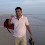## Tuesday, September 11, 2012

### Power Electronics Competitive Questions with Answers: Part 2

 The average output voltage(Vdc) of the full wave diode bridge rectifier is
(a) Vm/2
(b) 2Vm/π
(c) 3Vm/π
(d) 4Vm/π

 The typical ratio of latching current to holding current in a 20A thyristor is  [GATE -2011]
(a) 5.0
(b) 2.0
(c) 1.0
(d) 0.5

 A half controlled single phase bridge rectifier is supplying an R-L load. It is operated at a firing angle (alpha) and load current is continuous. The fraction of cycle that the freewheeling diode conducts is [GATE -2011]
(a)  1/2
(b)  [1-( α/π)]
(c)  α/2π
(d)  α/π

Common Data for Questions  & 
In the 3 phase inverter circuit shown, the load is balanced and the gating scheme is 180 degree conduction mode. All the switching devices are ideal , Vd  = 300VThe rms value of load phase voltage is [GATE -2011]

(a) 106.1V
(b) 141.4 V
(c) 212.2 V
(d) 282.8V

Hint: VL = (2/3) * Vs
 If the dc bus voltage Vd = 300v, the power consumed by 3 phase load is [GATE -2011]
(a) 1.5kW
(b) 2.0 kW
(c) 2.5 kW
(d) 3.0 kW

Hint: P = 3 * [(Vph * Vph)/Rph]
Common Data for Question 6 & 7:
10V Pulse Transformer (PT) is used to trigger the SCR in the adjacent figure. The SCR is rated at 1.5KV, 250A with IL=250mA, IH=150mA, and IGmax= 150mA, IGmin=100mA. The SCR is connected to an inductive load, where L=150mH in series with a small resistance and the supply voltage is 200V dc. The forward drops of all transistors/ diodes and gate- cathode junction during ON state are 1.0V.The resistance R should be
(a) 4.7K Ohm
(b) 470 Ohm
(c) 47 Ohm
(d) 4.7 Ohm

 The minimum approximate volt-second rating of the pulse transformer suitable for triggering the SCR should be: ( volt-second rating is the maximum of product of the voltage and the width of the pulse that may be applied
(a) 2000 μV-s
(b) 200 μV-s
(c) 20 μV-s
(d) 2.0 μV-s

 For an SCR with turn-on time of 5 μS, an ideal trigger pulse should have
(a) Short rise time with pulse width = 3 μS
(b) Long rise time with pulse width = 6 μS
(c) Short rise time with pulse width = 6 μS
(d) Long rise time with pulse width = 3μS

 Turn-on time for an SCRs is 10μS. If an inductance is inserted in the anode circuit, then the turn on time will be
(a) 10μS
(b) less than 10μS
(c) More than 10μS

 On-state voltage drop across a thyristor used in a 250V supply system is of the order of
(a) 100 - 110V
(b) 240 - 250V
(c) 1 - 1.5V
(d) 0.5 - 1V

 In a SCR, ratio of latching current to holding current is
(a) 0.4
(b) 1.0
(c) 2.5
(d) 6.0

Hint: Usually the ratio is near to 3
4:51 AM

#### 1 comment:

1.